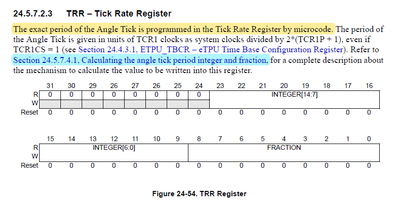# ETPU - Setting TRR in Engine Control

cancel
Showing results for
Did you mean:
SOLVED

## ETPU - Setting TRR in Engine Control

791 ViewsContributor III

Hi everyone,

I am currently working on eTPU - AN4907 Engine Control demo. In the etpuc_crank.c (CRANK function), I saw the below function.I understand the purpose of the function and I know we should set TRR for every tooth after the synchronization. However, I am struggling the understanding why we are shifting the mach register left by 9.

The below image shows the TRR register of the eTPU for MPC5644A microcontroller.In order to make clear everything, I want to give an example which the values are taken from the random point of the demo.

tooth_period = 272024 (in TCR1)

crank_local.tcr2_ticks_per_tooth = 100 (default value in demo)

First, we should process the "integer part of TRR";

1. tooth_period / crank_local.tcr2_ticks_per_tooth = 272024 / 100 = 2720 (rounding down - MACH register will be equal to the 24 which is called as remainder)
2. After the division in order to put the 2720 (integer part) to related bit field in the TRR register we should shift it left by 9. => 2720 << 9 = 1392640 (0x154000 in hex)

Secondly, we should process the "fractional part of TRR";

1. mach (which is equal to the 24) << 9 = 12288
2. After the shifting mach register, in order to convert the remainder to the fraction we divide the shifted mach with crank_local.tcr2_ticks_per_tooth => 12288 / 100 = 122 (0x7A in hex - rounding down)

Lastly, add them to get TRR value => 0x154000 + 0x7A = 0x15407A

Normally, if we do NOT work on bits, I should expect the presentation of the fraction part of TRR is 24/100 where it means that mach / crank_local.tcr2_ticks_per_tooth. As you can notice, there is no shifting in the mach! However in aspect of bits, if divide 24 to 100, we will get 0 (zero). So, this is not gonna be work.

So, why we are shifting mach (remainder) by 9 where I mark it with red color? Is this related with the design of the eTPU?

I tried to explain more clearly, hope you understand what I ask. Also, I read 24.5.7.4.1 Calculating the angle tick period integer and fraction section in RM of MPC5644A.

Best regards,

Mert.

Tags (5)
1 Solution
785 ViewsContributor III

Oh, I've struggle that question for 2-3 days and when I checking my question. I noticed something

Again, we assume that, tooth_period = 272024 and crank_local.tcr2_ticks_per_tooth = 100. In the integer part, we are shifting the division result left by 9. But, what is the result value of division? It is 2720 (tooth_period / crank_local.tcr2_ticks_per_tooth). This number can be found also if the tooth_period = 272000. I mean, we are losing the effect of the last two digit (two digit beucase we divide with 100) of the tooth period. Because we didn't shift the tooth_period, we shift the result of the division!

Therefore, in order to preserve the importance of the value of 24 (actually it's remainder right ), we should shift the remainder left by 9! Then we can divide it again the crank_local.tcr2_ticks_per_tooth to get raction part of the trr.

I hope it is understandable. If I am true, I would like to know that, so I am still looking forward for your helps friends.

Best regards,

Mert.

6 Replies
585 ViewsContributor III

Hi @mertk ,

Can you please let me know where to obtain "etpuc_crank.c" file. all i can find in AN4907SW is "etpu_crank.c".

Thanks,

Arjun

564 ViewsContributor III

https://www.ashware.com/Software

Best regards,

Mert.

543 ViewsContributor III

hello @mertk ,

Thanks for the resources.

763 ViewsContributor III

Mert.

786 ViewsContributor III

Oh, I've struggle that question for 2-3 days and when I checking my question. I noticed something

Again, we assume that, tooth_period = 272024 and crank_local.tcr2_ticks_per_tooth = 100. In the integer part, we are shifting the division result left by 9. But, what is the result value of division? It is 2720 (tooth_period / crank_local.tcr2_ticks_per_tooth). This number can be found also if the tooth_period = 272000. I mean, we are losing the effect of the last two digit (two digit beucase we divide with 100) of the tooth period. Because we didn't shift the tooth_period, we shift the result of the division!

Therefore, in order to preserve the importance of the value of 24 (actually it's remainder right ), we should shift the remainder left by 9! Then we can divide it again the crank_local.tcr2_ticks_per_tooth to get raction part of the trr.

I hope it is understandable. If I am true, I would like to know that, so I am still looking forward for your helps friends.

Best regards,

Mert.

770 ViewsContributor IV

I believe your description is accurate.  The initial tooth period cannot be shifted left before the first division because in many cases (slower engine speeds) overflow would result, thus the decomposition into two divides.

John Diener